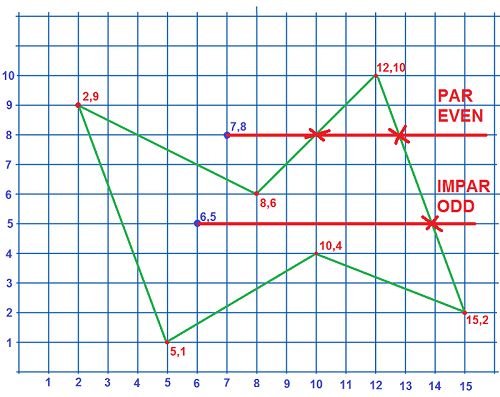# Geofence. Check if a point is inside a polygon. JavaScript. Map

Geo-fence. Check if a point is inside a polygon.- JavaScript:

``````// var poligono = [[2,9],[8,6],[12,10],[15,2],[10,4],[5,1]];
// var punto = [6, 5];

function pointInPolygon(polygon, point) {
let odd = false;
for (let i = 0, j = polygon.length - 1; i < polygon.length; i++) {
if (((polygon[i] > point) !== (polygon[j] > point))
&& (point < ((polygon[j] - polygon[i]) * (point - polygon[i]) / (polygon[j] - polygon[i]) + polygon[i]))) {
odd = !odd;
}
j = i;
}
return odd;
};

result = pointInPolygon(poligono, punto);
AppInventor.setWebViewString(result);
``````
• I have used poligono and punto as variable names.
4 Likes

2.- Check if a point is inside a polygon. JavaScript. RunJavaScript.

p251_geofenceJavaScript.aia (165.3 KB)

- Designer.

- Blocks.

• The code returns a String "true" or "false".
• Be careful with the semicolons;
• I have used poligono and punto as variable names.

3.- Map. Check if a point is inside a polygon on a map. Geofence.

p251_geofenceJavaScript_Mapa.aia (4.4 KB)

• We draw a polygon in a Map using LineString.PointsFromString, note that the initial and final coordinates are the same so that the polygon closes:

LineString.PointsFromString=[[36.50318,-6.27281],[36.50226,-6.27231],[36.50198,-6.27311],[36.50291,-6.27361],[36.50263,-6.27298],[36.50318,-6.27281]]

• We set the check polygon with these coordinates:

poligono = [[36.50318,-6.27281],[36.50226,-6.27231],[36.50198,-6.27311],[36.50291,-6.27361],[36.50263,-6.27298]]

• We move by means of a Clock, a point horizontally:

punto = [36.5027,h]

• We can stop the Clock using "Stop" and tap on the map, using the TapAtPoint block we can see if the point is inside or outside the polygon.

- Designer.

- Blocks.

• It also works if we set the query polygon by repeating the initial coordinate at the end:
poligono = [[36.50318,-6.27281],[36.50226,-6.27231],[36.50198,-6.27311],[36.50291,-6.27361],[36.50263,-6.27298],[36.50318,-6.27281]]

• The code returns a String "true" or "false".

4.- Extension Geofence.

• Instead of creating the code using JavaScript, we are going to create it with Java to make an extension, we will see it in this topic:

Part of this example can be accomplished more simply without javascript using a Polygon component.

geofenceSimpleSteveJG.aia (3.2 KB)

...just another way.You present an interesting alternative.

1 Like